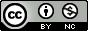Scientific journal NRU ITMO
Series "Refrigeration and Air Conditioning"
Registration certificate ЭЛ № ФС 77 – 55410 от 17.09.2013
зарегистрировано в Федеральной службе по надзору в сфере связи, информационных технологий и массовых коммуникаций
ISSN:2310-1148
Publications

June 2015 (published: 28.05.2015)

Number 2(18)

Home > Issue > The Maxwell construction to search thermodynamic values at vapour pressure curve

## The Maxwell construction to search thermodynamic values at vapour pressure curve

In the calculation of thermodynamic properties of substances on the vapour pressure curve you must consider the phase transition. As is known, the condition of equilibrium phases is the equality of their specific thermodynamic potentials. It is convenient to use the Maxwell construction. For equations of state, expressed in an analytical form, it is convenient to use the Maxwell construction. The Maxwell construction allows for this equation of state to find a vapour pressure curvep=p(T) or T=T(p) and specific volumes of liquid and vapour. This article is formulated and derived the Maxwell construction in a convenient form for further.  The Maxwell construction is composed of two systems of equations for finding thermodynamic quantities on the vapour pressure curve p=p(T) or T=T(p). Then the obtained results are applied to specific models of thermodynamic systems.
Read the full articleKeywords: the Maxwell construction, vapour pressure curve, liquid phase, gaseous phase.